# Get A Little Help With Calculus Thanks To These Awesome Apps

Learning calculus is definitely not easy. With so many calculations it's no wonder that the average calculous student would need a little extra help. That's where this collection of calculous apps comes in. Get help with pre calculus all the way up to more complex levels thanks to these awesome apps.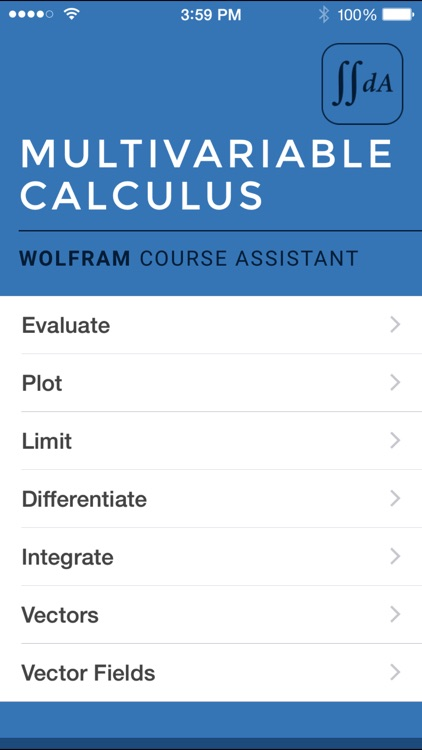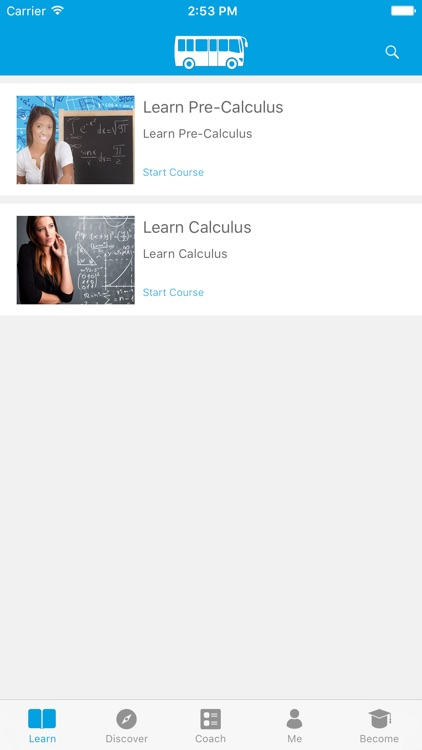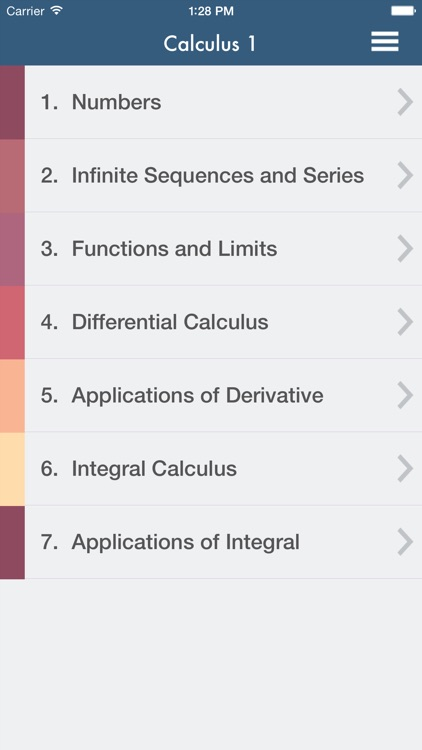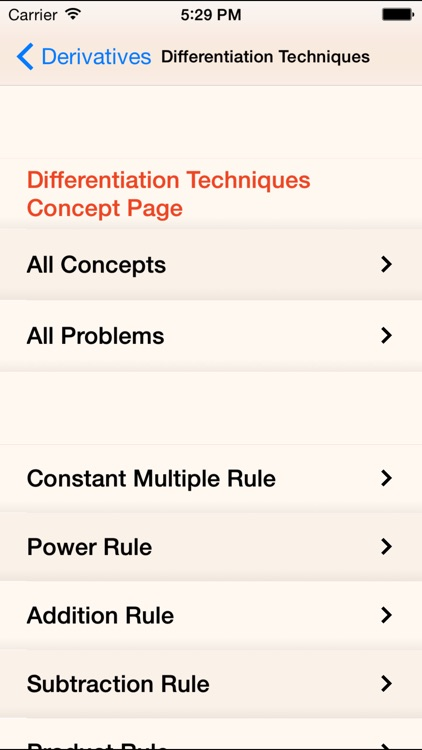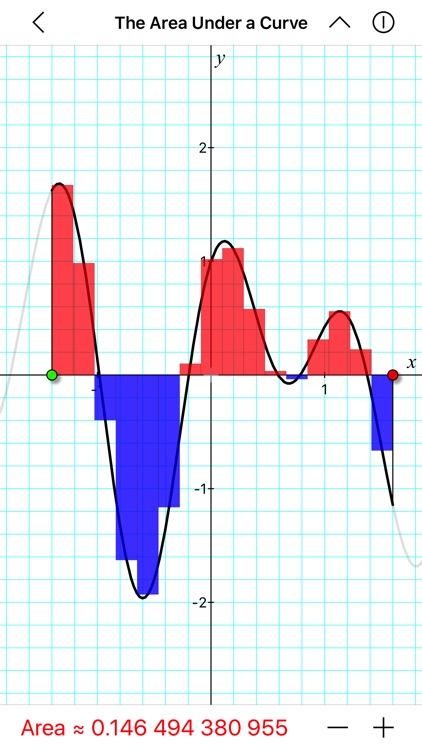## Best Apps

Highest scored apps in the category### Wolfram Multivariable Calculus Course Assistant

Wolfram Group LLC

Taking multivariable calculus

Taking multivariable calculus? Then you need the Wolfram Multivariable Calculus Course Assistant. This definitive app for multivariable calculus—from the world leader in math software—will help you work through your homework problems, ace your tests, and learn calculus concepts. Forget canned examples! The Wolfram Multivariable Calculus Course Assistant solves your specific multivariable problems on the fly, providing step-by-step guidance for limits,...### Learn Pre

Quizmine.Com

Learn “Pre-Calculus and Calculus” from AI driven coach and satisfy your thirst for knowledge

Learn “Pre-Calculus and Calculus” from AI driven coach and satisfy your thirst for knowledge.

App offers bite sized videos, quizzes and AI driven coach to help you become smarter and become great.

Just 60 minutes a week can help you become great in “Learn Pre-Calculus and Calculus”

Following chapters are available for rapid fast learning.

Entrepreneurship
MBA
Accounting
Marketing
Sales
Public Relations
...### Calculus 1

developWay CJSC

Doctor Math is really proud to introduce its first product, Calculus 1

Doctor Math is really proud to introduce its first product, Calculus 1.
The concept is very simple. Doctor Math is sure that learning mathematics becomes very effective, if the students apply their learned theory by solving as many practical problems as possible.

Doctor Math Calculus 1 offers the students more than 280 practical problems. All solutions of problems and their detailed explanations...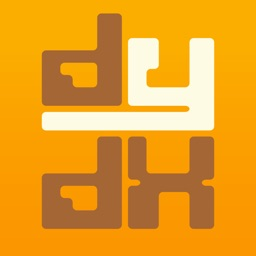### Calculus FTW

MathFTW LLC

Does calculus have you dazed and confused

Does calculus have you dazed and confused? Calculus FTW
has hundreds of detailed step-by-step solutions to example problems from first year calculus!

Written by a college professor, Calculus FTW is an app that helps students learn calculus from a problem based approach.

Calculus FTW is not a mere reference for equations. Calculus FTW actually helps you learn and understand calculus by...

## Also Good

Apps with average score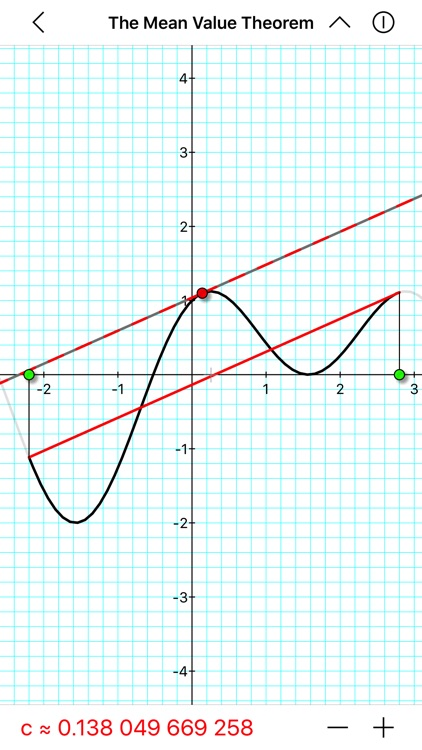### A Little Calculus

David Little

A LITTLE CALCULUS is a collection of more than 70 interactive topics from a first year calculus course. To be used by both teachers and students, this app is designed to aid in the teaching and learning of calculus and should serve as a nice supplement to any introductory calculus...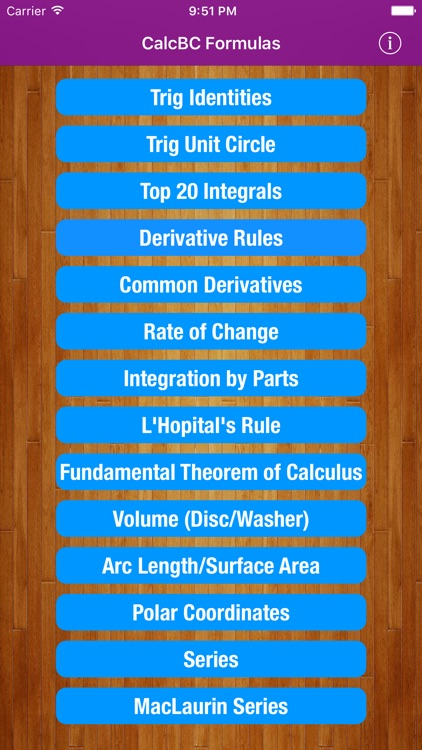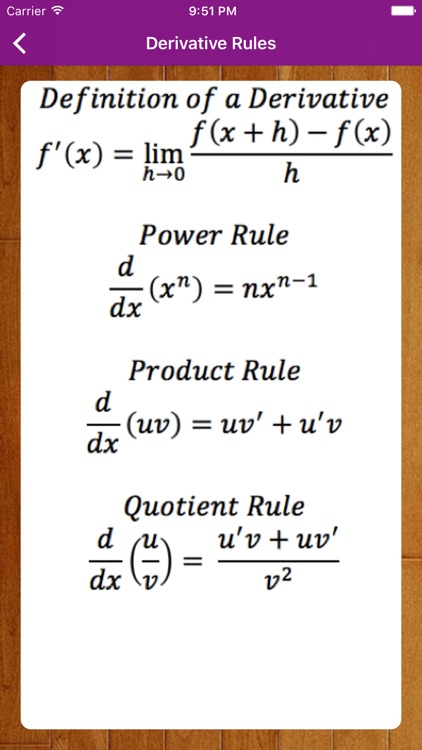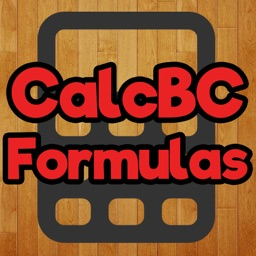### CalcBC Formulas

VinuIlangovan

This application was created to assist in Mathematics. It contains many useful formulas for those who are in Calculus AB (I) and Calculus BC (II). It is necessary to know all the formulas given in this app for the Calculus BC AP exam, but there are also other formulas not...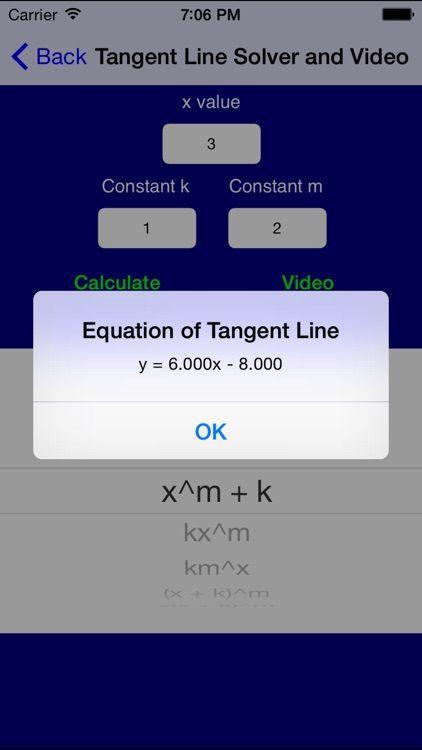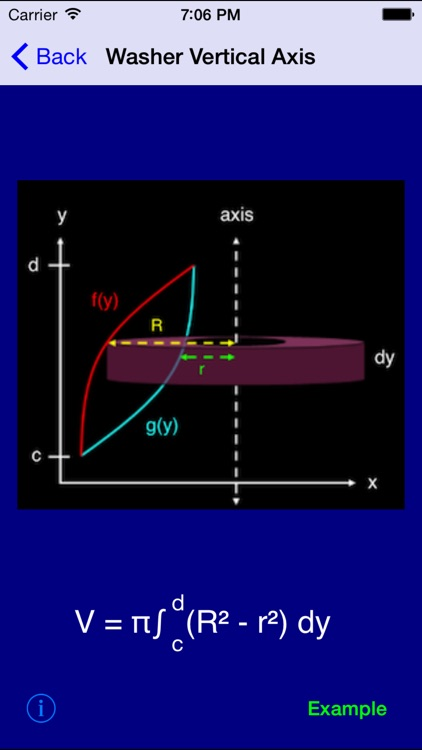### Calculus Pro

Larry Feldman

Calculus has been known to bring students to tears. Now you have an expert in your corner.

This application contains a rich collection of examples, tutorials, and solvers for the following topics:

- Limits
- Continuity
- Differentiability
- RDQ, LDQ, SDQ and NDQ
- Equations of Tangent Lines
- Partial Derivatives
- Implicit Differentiation
- Chain Rule
- Product...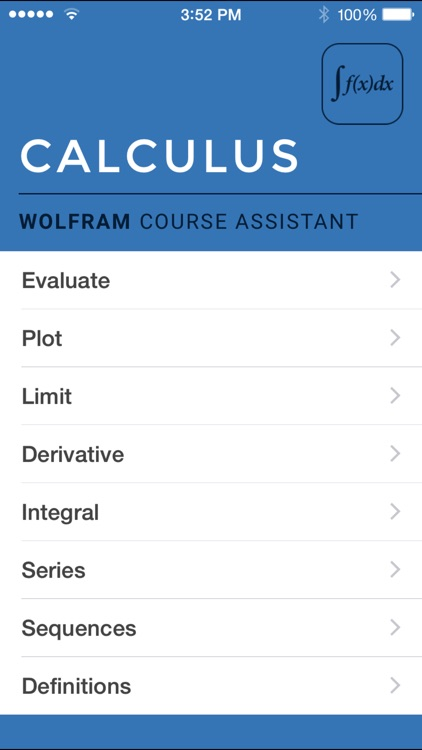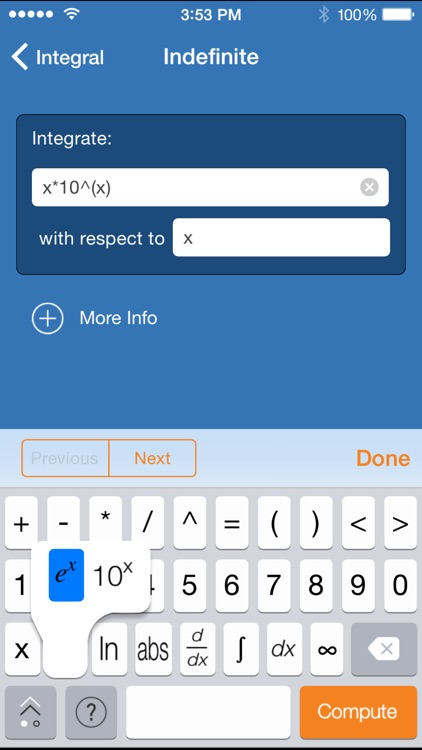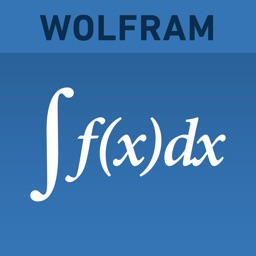### Wolfram Calculus Course Assistant

Wolfram Group LLC

Taking calculus? Then you need the Wolfram Calculus Course Assistant. This definitive app for calculus—from the world leader in math software—will help you work through your homework problems, ace your tests, and learn calculus concepts. Forget canned examples! The Wolfram Calculus Course Assistant solves your specific Calculus problems on the...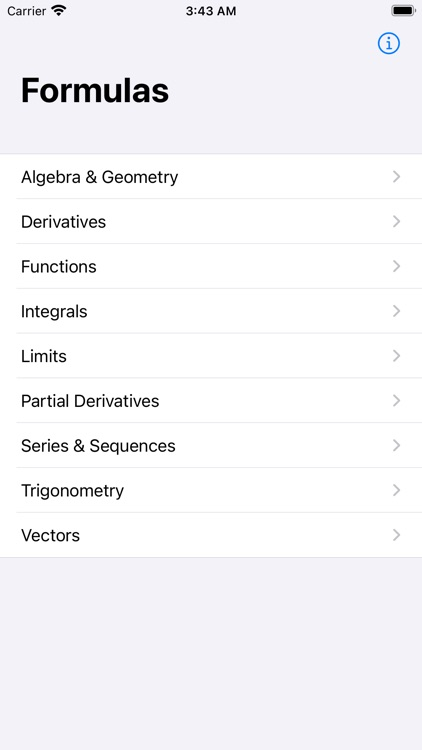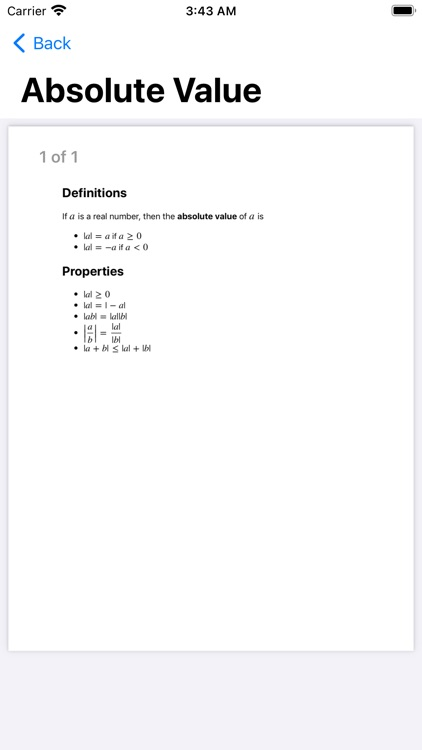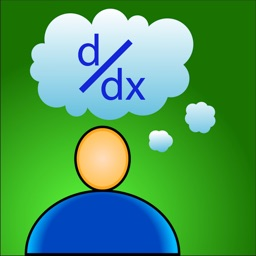### Formulus

Daniel Byon

Have you ever needed to know that one math formula that you always forget? Do you spend minutes flipping through your textbook or searching the internet for it? Then you need Formulus!

Formulus is the perfect study tool. It is a simple, easy to use, easy to navigate collection of...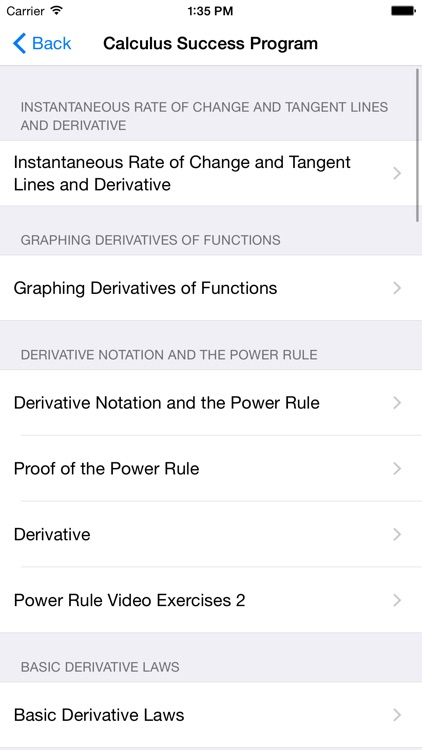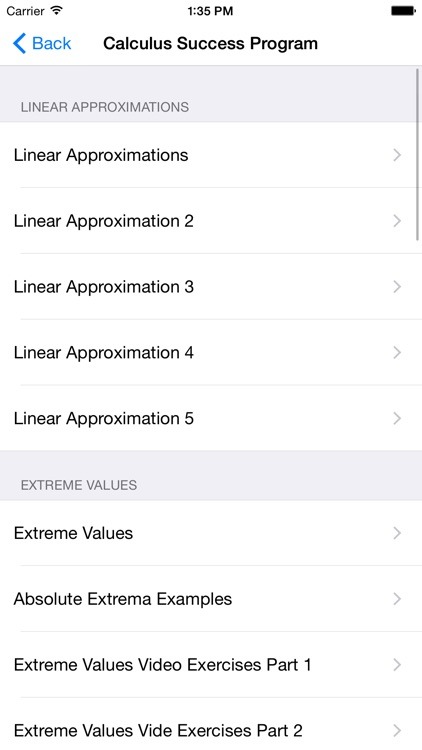### Calculus Success Program

Guillermo Alvarez

Access all of the www.calcsuccess.org videos for free on your iPhone or iPad. All of the videos for a typical Calculus 1 and 2 course are available!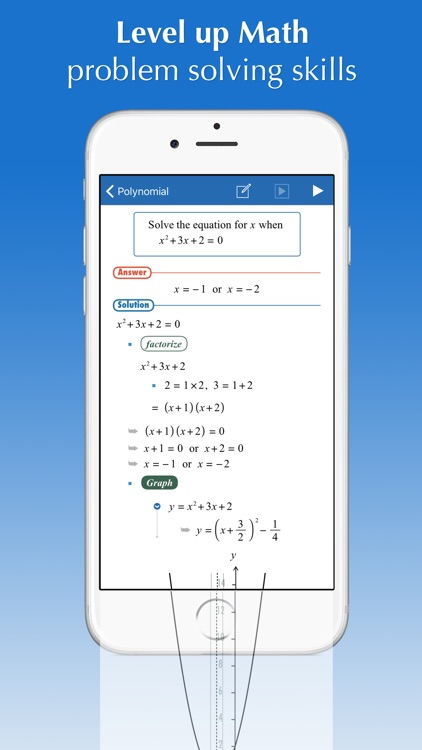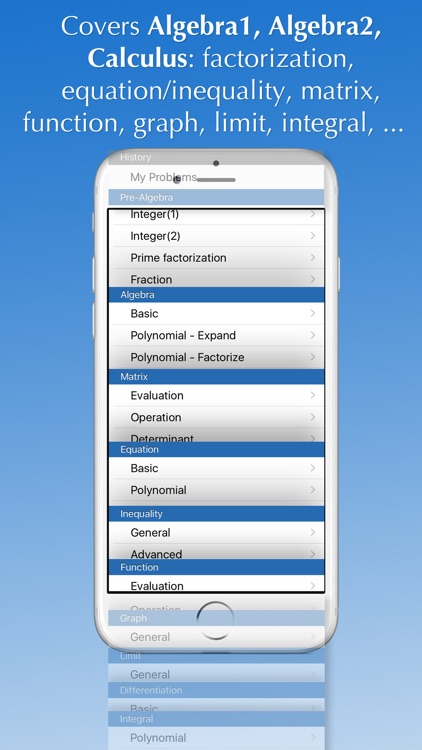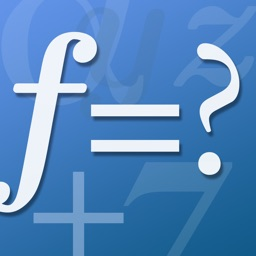### FX Math Solver

Euclidus Inc

Will guide you how to solve your math homework and textbook problems, anytime, anywhere.

FX Math Solver is a comprehensive math software, based on an automatic mathematical problem solving engine,
and ideal for students preparing term math exams, ACT, SAT, and GRE:

- Over 1,500 sample math problems and fully animated solution...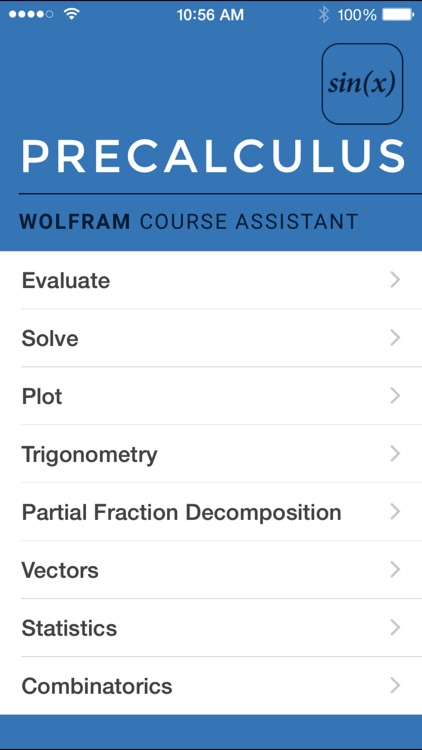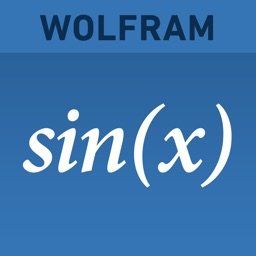### Wolfram Precalculus Course Assistant

Wolfram Group LLC

Taking precalculus? Then you need the Wolfram Precalculus Course Assistant. This definitive app for precalculus—from the world leader in math software—will help you work through your homework problems, ace your tests, and learn calculus concepts. Forget canned examples! The Wolfram Precalculus Course Assistant solves your specific precalculus problems on the...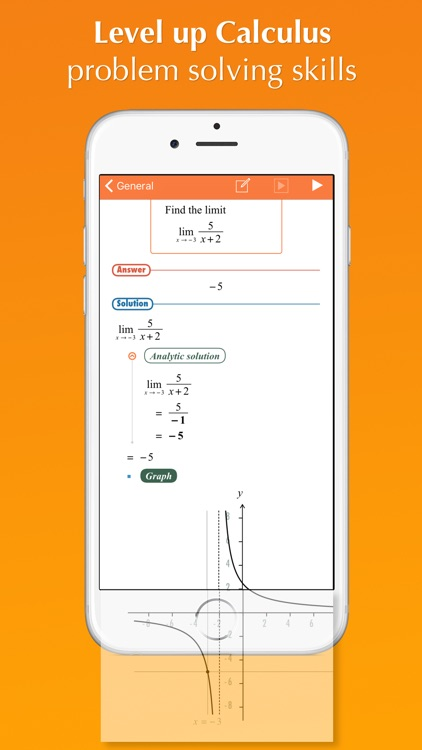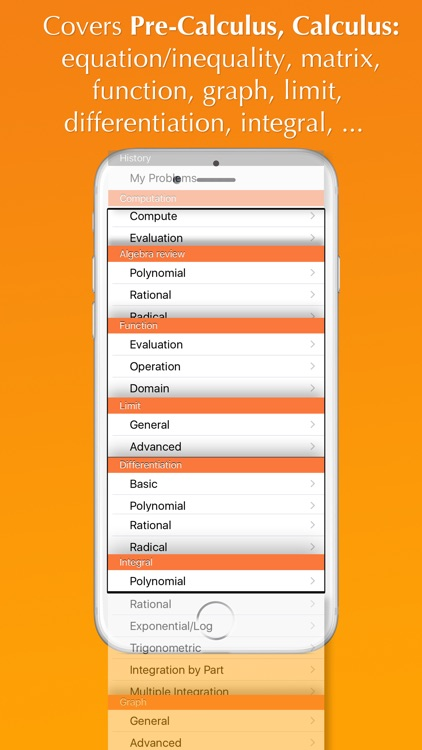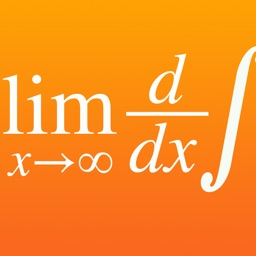### FX Calculus Problem Solver

Euclidus Inc

Will guide you how to solve your Calculus homework and textbook problems, anytime, anywhere.

FX Calculus Solver is a comprehensive math software, based on an automatic mathematical problem solving engine,
and ideal for students preparing term math exams, ACT, SAT, and GRE:

- Over 1,000 sample math problems and fully animated...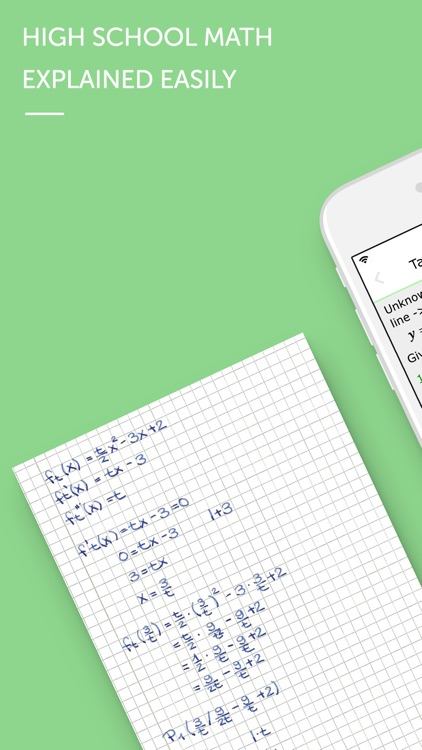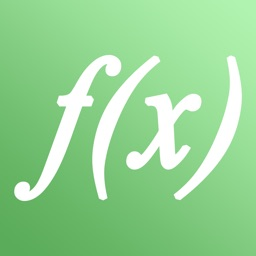### High School Math - Calculus

Graphing Calculator Apps UG (haftungsbeschrankt)

The perfect high school & college app for math (calculus, solving equations and matrices) which combines theory and practice!

6 reasons to buy this app:
1. Choose from 20 topics
2. Easy step by step explanations
3. Examples with numbers/parameters
4. Infoboxes include more details
5. Over 40 math problems to train
6. Exact handwritten solutions

More...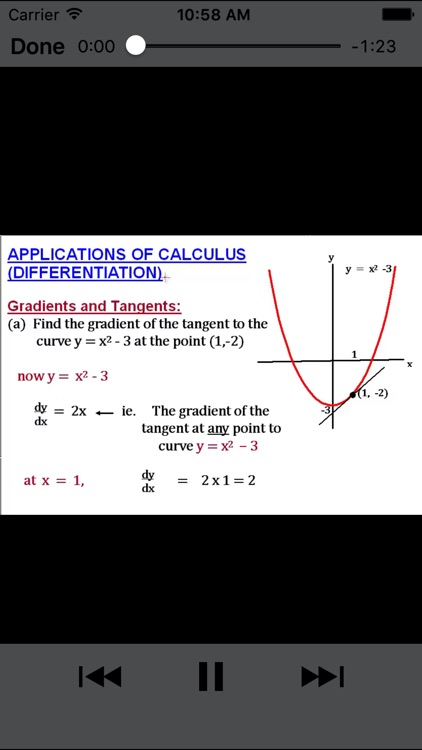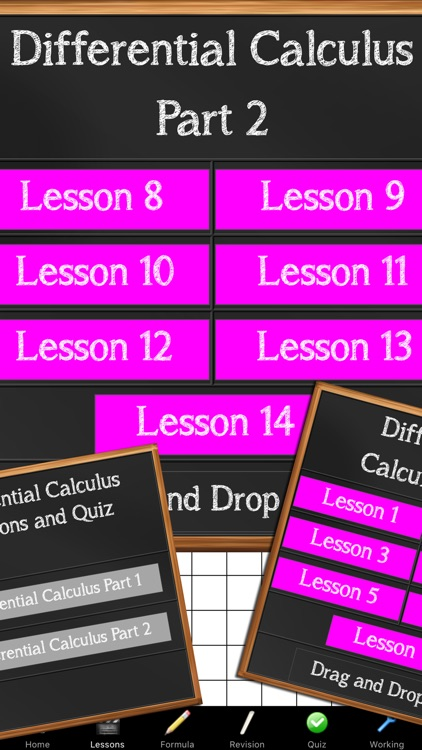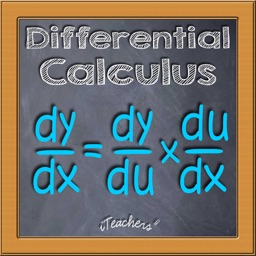### Differential Calculus Lessons

D.P STACE & G.J STACE & S.A WILLIAMS

Applications of Calculus (Differentiation) is an app for students wanting to master Calculus the easy way.

With our INTERNATIONAL content, EASY TO UNDERSTAND, FAST PACED lessons, YOU WILL SOON BE TOP OF THE CLASS and heading in the right direction to achieve your dream of a DYNAMIC CAREER.

The longer you delay...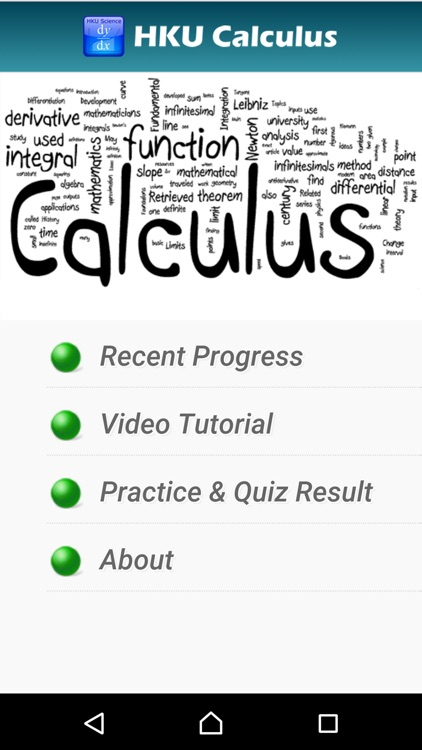### HKU Calculus

Chi Hong Chow

This app is a friendly introduction to Calculus. It is suitable for senior secondary students with little or no prior knowledge to Calculus. In this app, you will be introduced to functions, limits, differentiation, integration and differential equations. Each chapter contains videos and practice quizzes. The videos explain concepts and...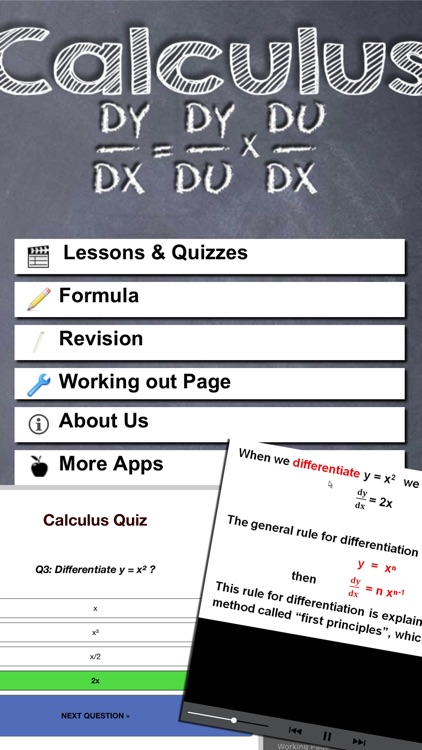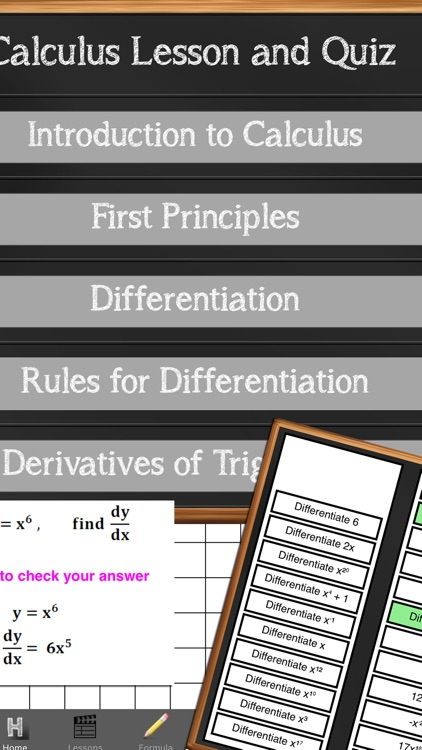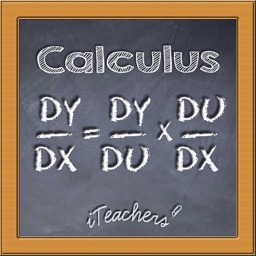D.P STACE & G.J STACE & S.A WILLIAMS

Calculus made Easy is an app for students wanting to master Calculus the easy way. Using the knowledge of a teacher who has taught for over 38 years with a Pure and Applied Mathematics Degree, you will find learning calculus a breeze.

Calculus made Easy has tutored lessons which shows...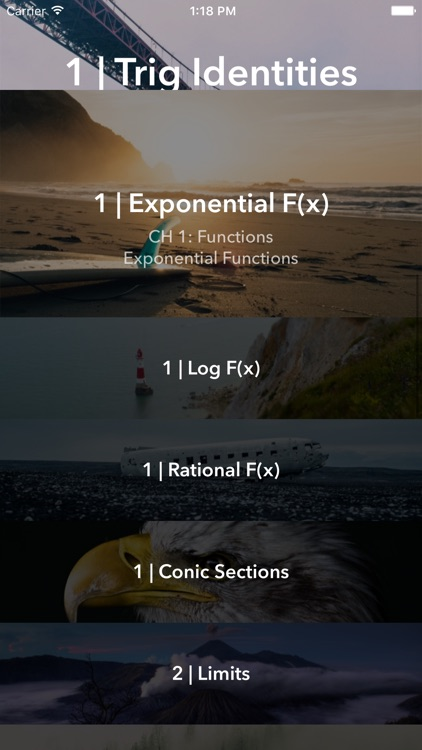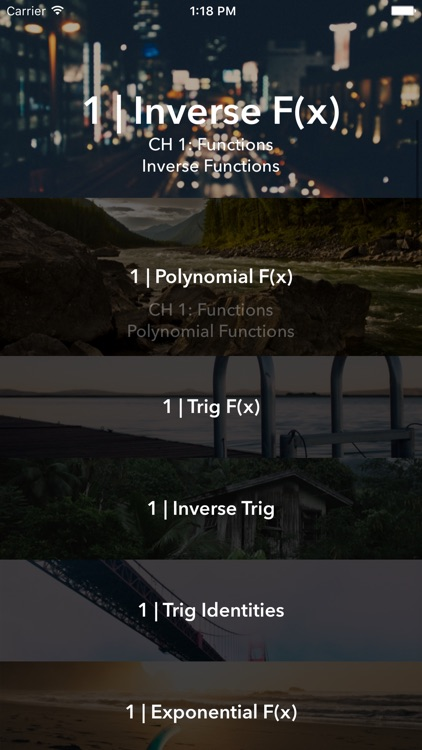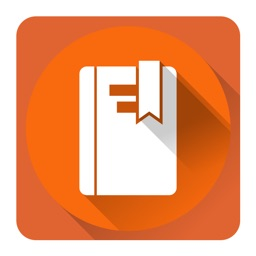### AP Calculus Complete Course (Calculus I)

Arshin Jain

New:
~~~~
Completely redesigned into an immersive layout which is not only appealing but is simple to use.

Apple TV Support—Now users may turn on Airplay to view content on Large TV Screen for added convenience.

We noticed the app crashed on certain devices and versions of iOS 9 and have eliminated all the...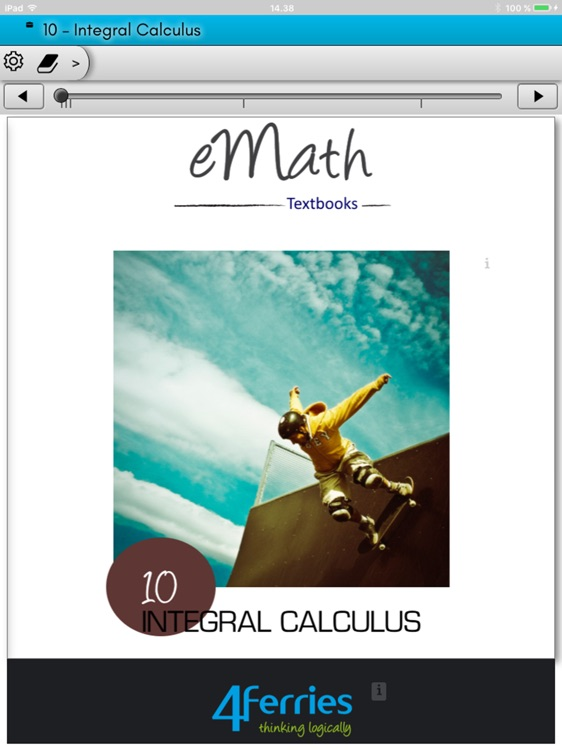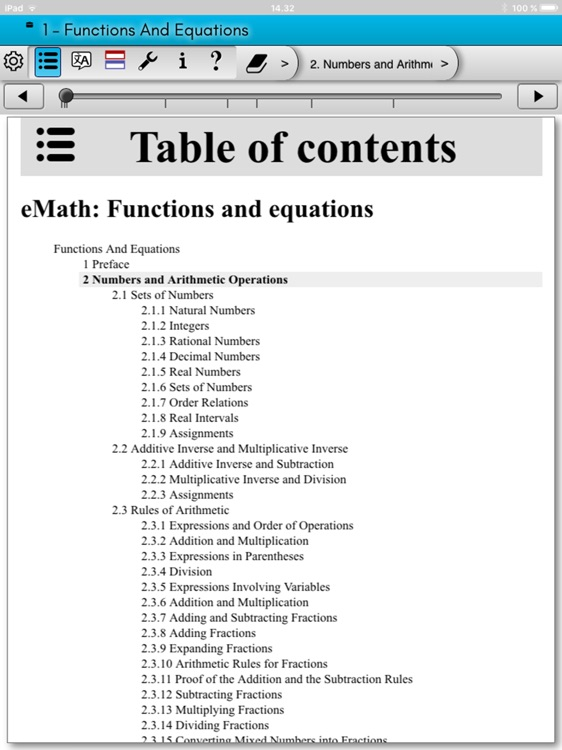### eMath10: Integral calculus

Four Ferries Ltd

eMath 10 - Integral Calculus

eMath 10 - Integral Calculus is a mathematics textbook for high school students and teachers. The book follows the Finnish national curriculum (2004) for mathematics education in high school. It can be used as supplementary material for standard mathematics courses in high school. The following topics...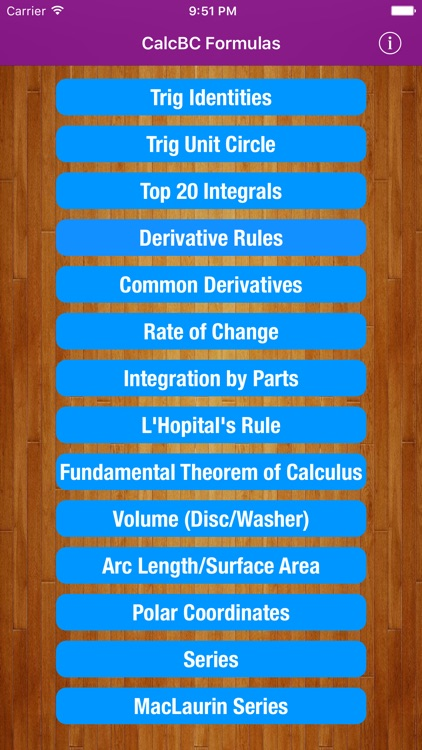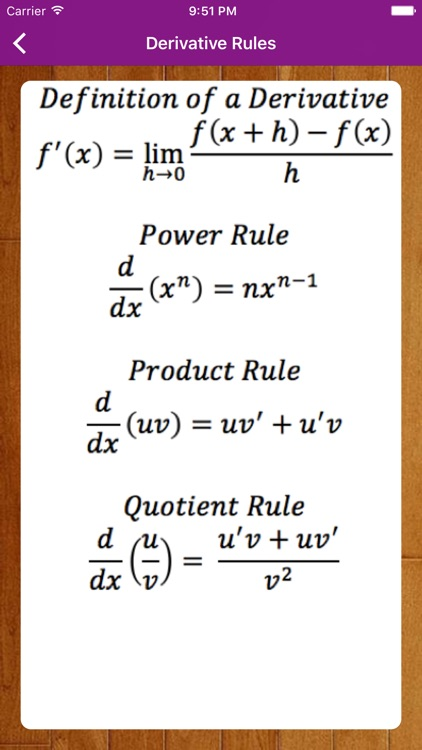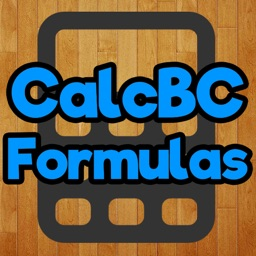### CalcBC Pro

VinuIlangovan

This application was created to assist in Mathematics. It contains many useful formulas for those who are in Calculus AB (I) and Calculus BC (II). It is necessary to know all the formulas given in this app for the Calculus BC AP exam,...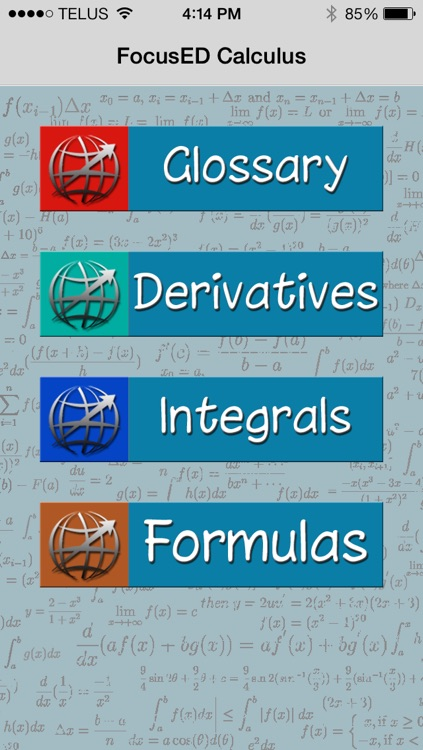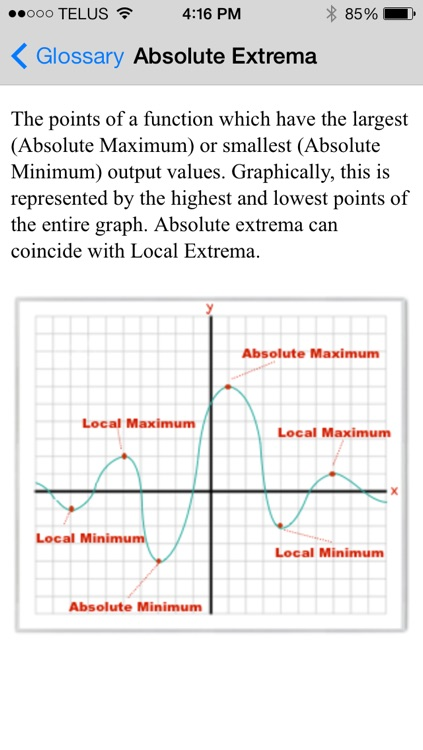### FocusED Calculus

Provectus Education

FocusED Calculus is the ultimate reference tool for calculus! If you are taking AP Calculus, Grade 12 Calculus or first year university calculus this reference guide is for you.

In this app you will find a glossary of over 300 calculus terms - many with illustrations or animations. Also included is...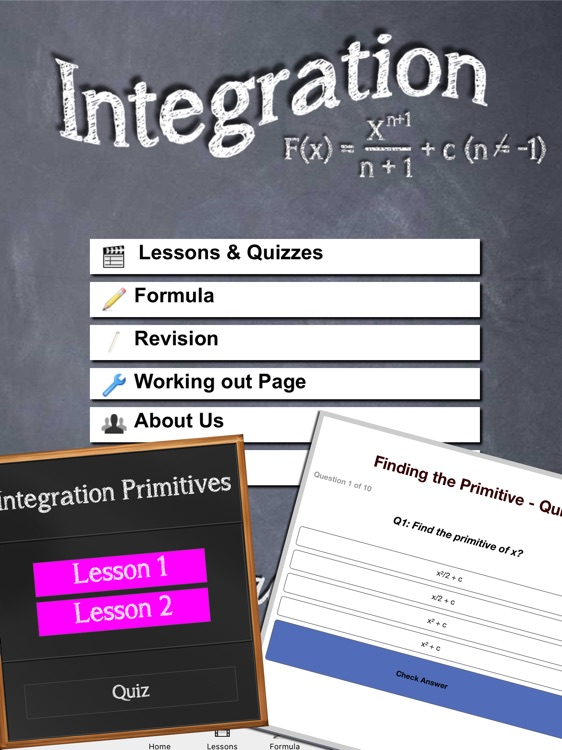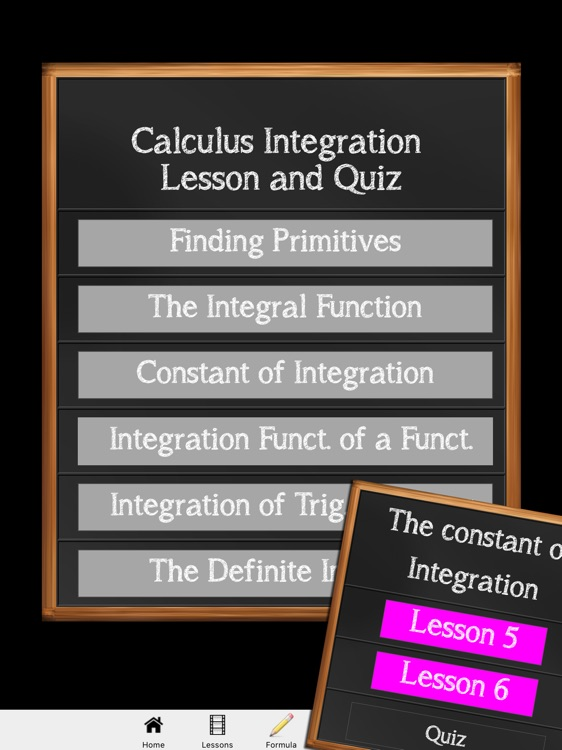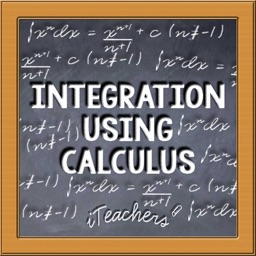### Integration Using Calculus

D.P STACE & G.J STACE & S.A WILLIAMS

Integration using Calculus is an app for students wanting to master Integration the easy way. Using the knowledge of a teacher who has taught for over 38 years with a Pure and Applied Mathematics Degree, you will find learning Integration a breeze.

For less than a cup of coffee, you will...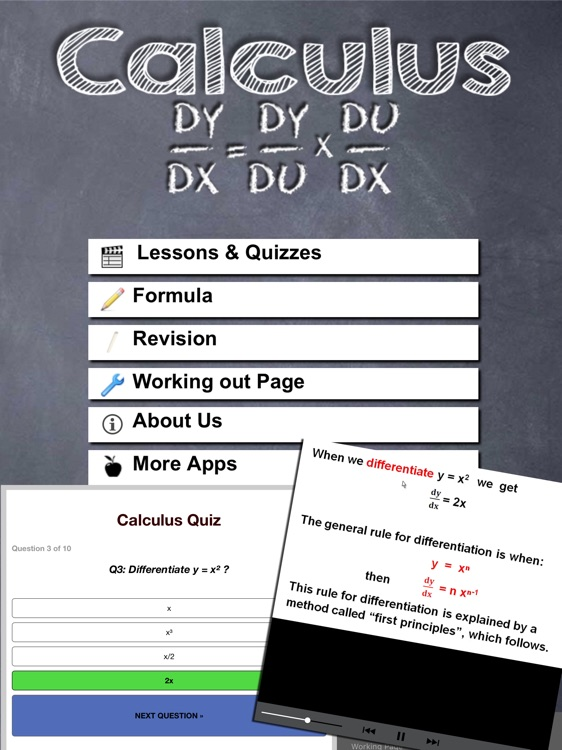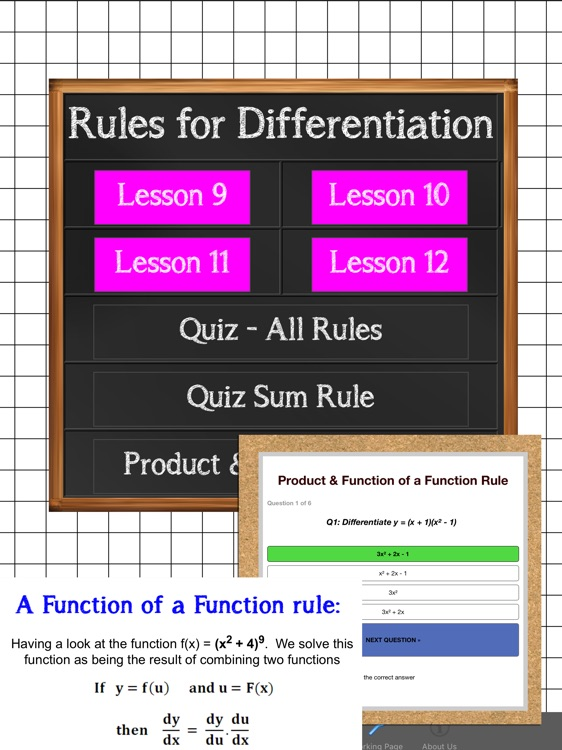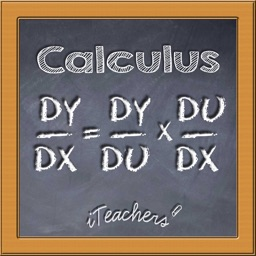### Calculus Maths

D.P STACE & G.J STACE & S.A WILLIAMS

Calculus made Easy is an app for students wanting to master Calculus the easy way. Using the knowledge of a teacher who has taught for over 38 years with a Pure and Applied Mathematics Degree, you will find learning calculus a breeze.

Calculus made Easy has tutored lessons which shows...

## Other Apps

Could be good for special cases